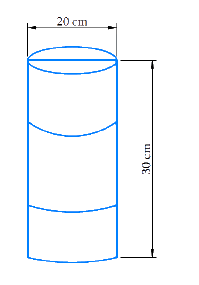# Ex.13.2 Q10 Surface Areas and Volumes - NCERT Maths Class 9

Go back to  'Ex.13.2'

## Question

In the below figure, you see the frame of a lampshade. It is to be covered with a decorative cloth. The frame has a base diameter of $$20 \,\rm{}cm$$ and height of $$30 \,\rm{}cm.$$ A margin of $$2.5 \,\rm{}cm.$$ is to be given for folding it over the top and bottom of the frame. Find how much cloth is required for covering the lampshade.Video Solution
Surface-Areas-And-Volumes
Ex exercise-13-2 | Question 10

## Text Solution

Reasoning:

The curved surface area of a right circular cylinder of base radius r and height h is and its total surface area \begin{align}2\pi r(r + h) \end{align}.The amount of cloth required to cover the cylinder will be equal to surface are of cylinder. (Here height will will equal to the height of cylinder plus $$2.5 \,\rm{}cm.$$ margin on both sides).

What is the known?

The height and diameter of the frame of lampshade. And the margin of $$2.5 \,\rm{}cm.$$ for folding.

What is the unknown?

Cloth required for covering the lamp shade.

Steps:

Diameter $$= 2r = 20\,\rm{}cm$$

$$r = 10 \rm{}\,cm$$

Height of the lamp shade $$= 30\,\rm{} cm + 2.5 \,\rm{}cm$$ for both side folding

Total height of the cloth $$= 35 \,\rm{}cm$$

Cloth required is the curved surface area

\begin{align}&=2\pi rh\\ &= 2 \times \frac{{22}}{7} \times 10 \times 35\\ &= 2200\,\,\rm{c{m^2}} \end{align}

Cloth required \begin{align} = 2200\,\,\rm{{cm^2}} \end{align}

Learn from the best math teachers and top your exams

• Live one on one classroom and doubt clearing
• Practice worksheets in and after class for conceptual clarity
• Personalized curriculum to keep up with school